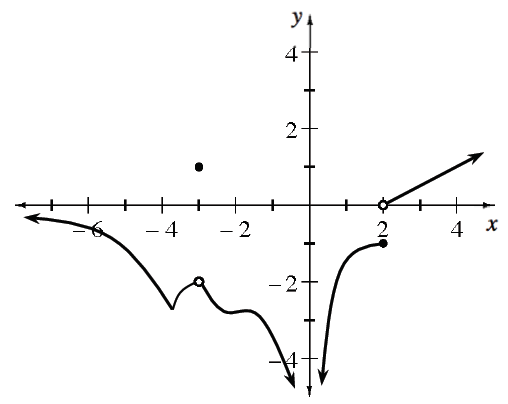### Home > APCALC > Chapter 3 > Lesson 3.1.2 > Problem3-29

3-29.

Is the function graphed below continuous at the following values of $x$? If not, explain which conditions of continuity fail.

$x = –4, –3, 0,$ and $2$

Three conditions of continuity:

$1. \lim \limits_{x \rightarrow a} f(x) \text{ exists} \\ 2. f(a) \text{ exists}\\ 3. \lim \limits_{x \rightarrow a} f(x) =f(a)$